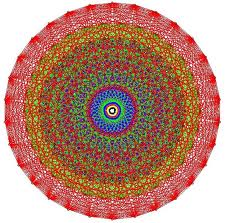### YÜRÜTÜCÜLER

Prof. Dr. Teoman ÖZER### ARAŞTIRMACILAR

Araş Gör. Gülden GÜN POLAT
Araş. Gör. Özlem ORHAN
Mecvut Laboratuvar İmkanları
Başlıca Çalışmalar ve Projeler
Ticarileşen, Ticarileşme Aşamasında olan veya Ticarileşme Potansiyeli olan Ürünler
Geliştirilen Başlıca Yöntemler, Prosedürler, Mekanizmalar, Ürünler, Yönetmelikler
Yayınlar, Patentler vb.

Journal publications

1. T. Özer, 2013 (with A. F. Cheviakov, S. Weichen, N. Migranov, T. R. Sekhar, and E. Yaşar), Editorial, Advances in Lie Groups and Applications in Applied Sciences, Abstract and Applied Analysis.

2. T. Özer, 2013 (with G. Gün), First integrals, integrating factors and invariant solutions of the path equation based on Noether and Lambda-symmetries, Abstract and Applied Analysis.

3. T. Özer, 2013 (with Ö. Orhan and G. Gün), On symmetry group classification of fin equation, Journal of Inequalities and Applications, 147.

4. T. Özer, 2012 (with V. B. Taranov, R. G. Smirnov, T. Klemas, P. Thamburaja, S. Wijesinghe, and B. Polat), Editorial, Recent Advances in Analytical Methods in Mathematical Physics, Advances in Mathematical Physics.

5. T. Özer, 2011 (with F. Rezvan and E. Yasar), Group properties and conservation laws for nonlocal shallow water wave equation, Applied Mathematics and Computation, 218, 974-979.

6. T. Özer, 2011 (with E. Yasar), On symmetries, conservations laws and similarity solutions of foam drainage equation, International Journal of Non-Linear Mechanics, 46, 357-362.

7. T. Özer, 2011 (with E. Yasar), Application of the composite variation principle to shallow water equations, Nonlinear Science and Complexity, 73-78.

8. T. Özer, 2010 (with D. Sahin), Theoretical-group analysis of the inviscid gravity currents, Journal of Interdisciplinary Mathematics, 13, 355-376.

9. T. Özer, 2010 (with E. Yasar), On the conservation laws and traveling wave solutions to the BBM equation, Journal of Interdisciplinary Mathematics, 13, 77-86.

10. T. Özer, 2010 (with F. Rezvan), Invariant solutions of integro-differential Vlasov-Maxwell equations in Lagrangian variables by Lie group analysis, Computers & Mathematics with Applications, 59, 3412-3437.

11. T. Özer, 2010 (with E. Yasar), Invariant solutions and conservation laws to nonconservative FP equation, Computers & Mathematics with Applications, 59, 3203-3210.

12. T. Özer, 2010 (with E. Yasar), Conservation laws for one-layer shallow water wave systems, Nonlinear Analysis: Real World Applications, 11 (2), 838-848.

13. T. Özer, 2010 (with D. Sahin and N. Antar), Lie group analysis of gravity currents, Nonlinear Analysis: Real World Applications, 11 (2), 978-994.

14. T. Özer, 2009, New traveling wave solutions to AKNS and SKdV Equations, Chaos, Solitons & Fractals, 42, 577–583.

15. T. Özer, 2009, The Lie algebra of point symmetries of nonlocal collisionless Boltzmann equation in terms of moments, Chaos, Solitons & Fractals, 40, 793-802.

16. T. Özer, 2009, New exact solutions to CDF equations, Chaos, Solitons & Fractals, 39, 1371–1385.

17. T. Özer, 2008, Symmetry group analysis and similarity solutions of variant nonlinear long –wave equations, Chaos, Solitons & Fractals, 33, 722-730.

18. T. Özer, 2008, An application of symmetry groups to nonlocal continuum mechanics, Computers & Mathematics with Applications, 55, 1923-1942.

19. T. Özer, 2008 (with N. Antar), The similarity forms and invariant solutions of two-layer shallow-water equations, Nonlinear Analysis: Real World Applications, 9 (3), 791-810.

20. T. Özer, 2007, The group-theoretical analysis of nonlocal Benney equation, Reports on Mathematical Physics, 60 (1), 13-37.

21. T. Özer, 2005, Symmetry group analysis of Benney system and an application for the shallow-water equations, Mechanics Research Communications, 2 (3), 241-254.

22. T. Özer, 2005 (with S. S. Akhiev), Symmetry groups of the equations with nonlocal structure and an application for the collisionless Boltzmann equation, International Journal of Engineering Science, 43(1-2), 121-137.

23. T. Özer, 2004 (with E. G. Cravalho), On developments in interactive web-based learning modules in a thermal-fluids engineering course: Part II, International Journal of Engineering Education, 20(5), 849-860.

24. T. Özer, 2004, On symmetry group properties and general similarity forms of the Benney equations in the Lagrangian variables, Journal of Computational and Applied Mathematics, 169(2), 297-313.

25. T. Özer, 2003, Symmetry group classification for two-dimensional elastodynamics problems in nonlocal elasticity, International Journal of Engineering Science, 41(18), 2193-2211.

26. T. Özer, 2003, Symmetry group classification for one-dimensional elastodynamics problems in nonlocal elasticity, Mechanics Research Communications, 30(6), 539-546.

27. T. Özer, 2003, Solutions of Navier equations of classical elasticity using Lie symmetry groups, Mechanics Research Communications, 30(2), 193-201.

28. T. Özer, 2003 (with M. Kenworthy, J. G. Brisson, E. G. Cravalho and G. H. McKinley), On developments in interactive web-based learning modules in a thermal-fluids engineering course, International Journal of Engineering Education, 19(2), 305-315.

29. T. Özer, 2000, Solution of the Boussinesq problem using Lie symmetries, Mathematical and Computational Applications, 5(1), 1-11.

30. T. Özer, 1999, On the symmetry group properties of equations of nonlocal elasticity, Mechanics Research Communications, 26(6), 725-733.

Bilgi İşlem Daire Başkanlığı